# RFEM – Online Manual

Online manuals, introductory examples, tutorials, and other documentation.

# 6.8 Free Rectangular Loads

General description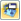A free rectangular load acts as a uniform or linearly variable surface load on a rectangular, freely definable zone of a surface.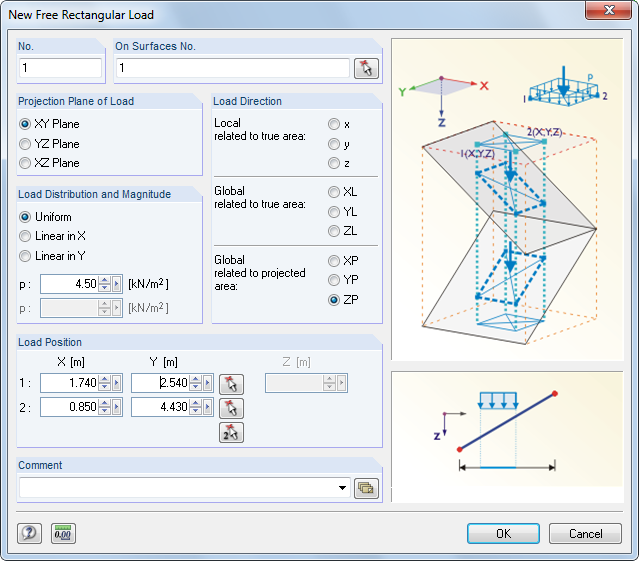Figure 6.38 New Free Rectangular Load dialog box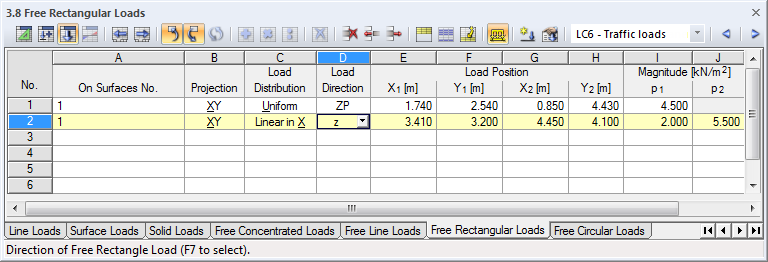Figure 6.39 Table 3.8 Free Rectangular Loads
On Surfaces No.

This text box manages the numbers of the surfaces on which the load acts. You can also select them graphically by using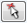.

Starting from the corner points defined by the Load Position, imaginary straight lines are "set" perpendicular to the projection plane. When these lines intersect any of the listed surfaces, the boundary line of the load that is to be applied is the connecting diagonal of both intersection points. In this way, it is possible to quickly allocate loads of a similar type to several surfaces.

Projection Plane

The load can be projected onto one of the global planes XY, YZ, or XZ. As described above, imaginary straight lines are generated, starting from both load positions and running perpendicular to the projection plane. The definition points of the rectangle are assumed wherever the imaginary straight lines intersect a surface.

The projection plane may not be perpendicular to a surface on which the load acts: There are no clear points of intersection with the surface.

Load Distribution

Specify whether a uniform or linearly variable force is applied. In the dialog text box below, you can enter one or two numerical values.

Load Direction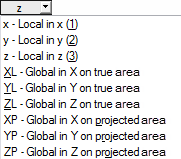The load can act in the direction of the local surface axes X, Y, Z or the global axes x, y, z.

• Local related to true area
• Loads that act perpendicular to the surface are usually defined as local in direction z.
• Global related to true area
• The orientation of the local surface axes is irrelevant for the calculation according to the linear static analysis if the load acts in the direction of an axis of the global coordinate system XYZ. An example of a load reference to the true area is self-weight.
• Global related to projected area
• The load is converted to the projection of the surface in one of the directions of the global coordinate system. A case of application is, for example, snow load. The graphic in the lower right of the dialog box illustrates the projected surfaces.
Load Position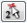Enter the coordinates of the load position into the text boxes. In the dialog box, you can also select the position of the load graphically by using thebutton.

Magnitude

Enter the numerical value of the area load in this table column or text box.